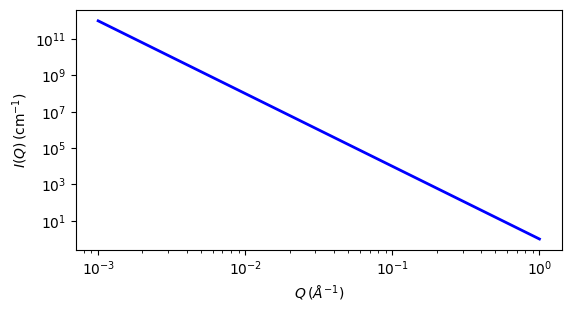# power_law

Simple power law with a flat background

Parameter

Description

Units

Default value

scale

Scale factor or Volume fraction

None

1

background

Source background

cm-1

0.001

power

Power law exponent

None

4

The returned value is scaled to units of cm-1 sr-1, absolute scale.

This model calculates a simple power law with a flat background.

Definition

$I(q) = \text{scale} \cdot q^{-\text{power}} + \text{background}$

Note the minus sign in front of the exponent. The exponent power should therefore be entered as a positive number for fitting.

Also note that unlike many other models, scale in this model is NOT explicitly related to a volume fraction. Be careful if combining this model with other models.Fig. 117 1D plot corresponding to the default parameters of the model.

Source

power_law.py

References

None.

Authorship and Verification

• Author: